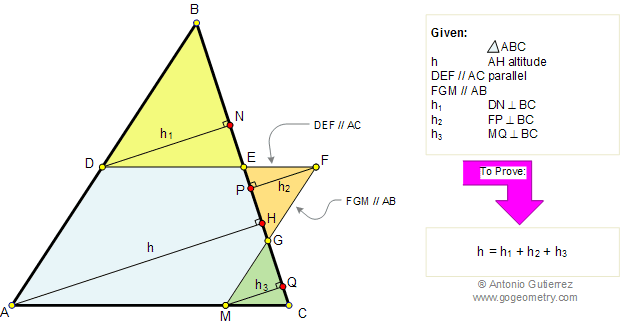# Problem 91. Similar Triangles, Altitude, Parallel, Perpendicular. Level: High School, College

 In the figure below, given a triangle ABC, an altitude AH = h, line DEF parallel to AC and line FGM parallel to AB. If h1, h2, and h3 are perpendicular to BC respectively, prove that: h = h1 + h2 + h3. View or post a solutionFACTS AND HINTS:

1. SIMILAR TRIANGLES:
Proposition:
Corresponding segments of similar triangles are in proportion.

Home | Geometry | Search | Problems | Altitude | All Problems | 91-100 | Email | View or post a solution | by Antonio Gutierrez